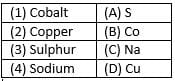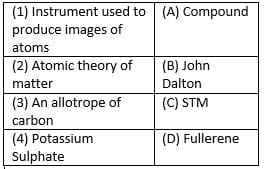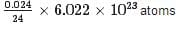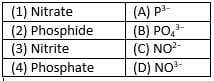UPSC  >  HOTS Questions: Atoms and Molecules

# HOTS Questions: Atoms and Molecules - UPSC

Test Description

## 25 Questions MCQ Test NCERT Textbooks (Class 6 to Class 12) - HOTS Questions: Atoms and Molecules

HOTS Questions: Atoms and Molecules for UPSC 2023 is part of NCERT Textbooks (Class 6 to Class 12) preparation. The HOTS Questions: Atoms and Molecules questions and answers have been prepared according to the UPSC exam syllabus.The HOTS Questions: Atoms and Molecules MCQs are made for UPSC 2023 Exam. Find important definitions, questions, notes, meanings, examples, exercises, MCQs and online tests for HOTS Questions: Atoms and Molecules below.
Solutions of HOTS Questions: Atoms and Molecules questions in English are available as part of our NCERT Textbooks (Class 6 to Class 12) for UPSC & HOTS Questions: Atoms and Molecules solutions in Hindi for NCERT Textbooks (Class 6 to Class 12) course. Download more important topics, notes, lectures and mock test series for UPSC Exam by signing up for free. Attempt HOTS Questions: Atoms and Molecules | 25 questions in 25 minutes | Mock test for UPSC preparation | Free important questions MCQ to study NCERT Textbooks (Class 6 to Class 12) for UPSC Exam | Download free PDF with solutions
 1 Crore+ students have signed up on EduRev. Have you?
HOTS Questions: Atoms and Molecules - Question 1

### Who suggested that if we go on dividing matter, a stage will come when particles obtained can’t be divided further?

Detailed Solution for HOTS Questions: Atoms and Molecules - Question 1

Democritus and Leucippus suggested that if we go dividing matter, a stage will come when particles obtain can’t be divided further.

HOTS Questions: Atoms and Molecules - Question 2

### Match the following with correct response.Detailed Solution for HOTS Questions: Atoms and Molecules - Question 2

Cobalt - Co
Copper - Cu
Sulphur - S
Sodium - Na
Hence, option B.

HOTS Questions: Atoms and Molecules - Question 3

### What information we get from molecular formula A It represents one molecule of the substance  B It does not tells the name of the substance  C It tells about the type of atoms  D It represents formula mass unit of one substance

Detailed Solution for HOTS Questions: Atoms and Molecules - Question 3
The chemical formula can tell you the amount of each individual element that makes up that compound. Usually, they are derived by mass, so if you multiply the subscript number by the elemental mass of the number preceding, you can find how much of that element is in that compound.
HOTS Questions: Atoms and Molecules - Question 4

One mole of N2 is equal to-

Detailed Solution for HOTS Questions: Atoms and Molecules - Question 4

B is the correct option.In order to have one mole of things, let's say particles, you need to have 6.022⋅1023 particles → this is known as Avogadro's constant and acts as the definition of the mole. So, in one mole of nitrogen gas you have 6.022⋅1023 molecules of nitrogen gas, N2

HOTS Questions: Atoms and Molecules - Question 5

Atom of Hydrogen is

Detailed Solution for HOTS Questions: Atoms and Molecules - Question 5

The diameter of Hydrogen atom is 10−10m. it is the smallest atom among all elemetns.

HOTS Questions: Atoms and Molecules - Question 6

Match the following with correct response.Detailed Solution for HOTS Questions: Atoms and Molecules - Question 6

STM ( scanning tunneling microscope)  is  an instrument for Imaging surfaces at the atomic level.
John Dalton gave the Atomic theory of Matter.
Fullerene is an Allotrope of carbon.
Potassium sulphate is a compound.

HOTS Questions: Atoms and Molecules - Question 7

How many moles are present in 40 g of He?

Detailed Solution for HOTS Questions: Atoms and Molecules - Question 7

Number of moles = mass of the substance molar mass. = 40/4 = 10 moles. Atomic mass or molar mass of Helium is 4 u.

HOTS Questions: Atoms and Molecules - Question 8

Calculate the number of Mg atoms in 0.024 g of Mg

Detailed Solution for HOTS Questions: Atoms and Molecules - Question 8

Atomic mass of Mg is 24. So, 24 g of Mg contains 6.022 x 1023 atoms. No. of atoms in 0.024 g of Mg == 6.022 x 1020 atoms.

HOTS Questions: Atoms and Molecules - Question 9

Who was the first to use the symbols for elements?

Detailed Solution for HOTS Questions: Atoms and Molecules - Question 9

Dalton was the first scientists who use the symbols for elements. The modern symbols are different from symbol proposed by Dalton.

HOTS Questions: Atoms and Molecules - Question 10

What according to Dalton’s atomic theory is true among the following-
A. Atom is divisible into Protons, electrons and neutrons
B. Atoms of the same element have different atomic masses.
C. Atoms are the ultimate indivisible particle of matter
D. Atoms of same element have same atomic masses size and chemical properties

Detailed Solution for HOTS Questions: Atoms and Molecules - Question 10

The correct answer is B as  all the statements of option c and d are true about dalton's theory .

HOTS Questions: Atoms and Molecules - Question 11

Which of the following elements are present in Quick lime?

Detailed Solution for HOTS Questions: Atoms and Molecules - Question 11

Chemical formula of Quick lime is CaO. So, elements present in Quick lime is Calcium and Oxygen.

HOTS Questions: Atoms and Molecules - Question 12

Statement A: mole is quite often known as chemists dozen
Statement B: the mass of one twelfth (1/12) of the mass of one atom of carbon taken as 1u.
Which of the two statement is true

Detailed Solution for HOTS Questions: Atoms and Molecules - Question 12

Mole is also known as chemist dozen. The mass of one twelfth of the mass of one atom of carbon taken as 1u. So, both the statements are correct.

HOTS Questions: Atoms and Molecules - Question 13

What is the Latin name of Potassium?

Detailed Solution for HOTS Questions: Atoms and Molecules - Question 13

Latin name of Potassium element is Natrium from which symbol of Sodium is derived as Na.

HOTS Questions: Atoms and Molecules - Question 14

During a chemical reaction, the sum of the masses of the reactants and products remains unchanged. This is known as

Detailed Solution for HOTS Questions: Atoms and Molecules - Question 14

Law of conservation of mass states that, during chemical reaction, the sum of the masses of the reactants and products remains unchanged.

HOTS Questions: Atoms and Molecules - Question 15

What is the formula of calcium phosphide?

Detailed Solution for HOTS Questions: Atoms and Molecules - Question 15

Chemical formula of Calcium phosphide is Ca3P2. Valency of Calcium is 2 and Phosphate is 3.

HOTS Questions: Atoms and Molecules - Question 16

How many molecules are present in one gram molecular mass of a substance?

Detailed Solution for HOTS Questions: Atoms and Molecules - Question 16

The number of molecules present in one gram molecular mass of a substance is 6.022 × 1023 molecules called as Avogadro number.

HOTS Questions: Atoms and Molecules - Question 17

How many atoms are present in PO4−3ion?

Detailed Solution for HOTS Questions: Atoms and Molecules - Question 17

There are 5 atoms in the given ion, in which 1 atom is of Potassium and 4 atoms of Oxygen.

HOTS Questions: Atoms and Molecules - Question 18

Statement A: Atoms can exist independently
Statement B: The law of constant proportions is applicable only to pure chemical compounds.
Which of the two Statements is true?

Detailed Solution for HOTS Questions: Atoms and Molecules - Question 18

An atom cannot exist independently. The law of constant proportions is applicable only to pure chemical compounds. So, only statement B is correct.

HOTS Questions: Atoms and Molecules - Question 19

What is the mass of 0.5 mole of Hydrogen atom

Detailed Solution for HOTS Questions: Atoms and Molecules - Question 19

Atomic mass of hydrogen is 1. So, one mole of Hydrogen is equal to 1 g. Mass of 0.5 mole of Hydrogen atom will be 0.5×1=0.5g.

HOTS Questions: Atoms and Molecules - Question 20

The atomic mass of calcium is 40 the number of moles in 60 g of calcium are

Detailed Solution for HOTS Questions: Atoms and Molecules - Question 20
Number of moles of Ca = Given mass of calcium / Molar mass of calcium = 60 / 40 = 1.5 moles.
HOTS Questions: Atoms and Molecules - Question 21

What is the name given to the short hand representation of an element?

Detailed Solution for HOTS Questions: Atoms and Molecules - Question 21

Short hand representation of an element is called symbol. Symbol of an element is derived from English name or Latin name.

HOTS Questions: Atoms and Molecules - Question 22

Which postulates of Dalton’s atomic theory is the basis of the law of conservation of mass?

Detailed Solution for HOTS Questions: Atoms and Molecules - Question 22

The Dalton’s postulates that Atoms can neither be created nor destroyed are the basis of law of conservation of masses.

HOTS Questions: Atoms and Molecules - Question 23

How many moles are present in 2.3 g of Na

Detailed Solution for HOTS Questions: Atoms and Molecules - Question 23

Molecular mass of Sodium is 23 u. So, 23 g of sodium is equal to 1 mole. The number of mole in 2.3 g = 2.3 / 23 = 0.1 mole.

HOTS Questions: Atoms and Molecules - Question 24

Match the following with correct response.Detailed Solution for HOTS Questions: Atoms and Molecules - Question 24

A is the correct option.
Nitrate is a polyatomic ion with the chemical formula NO3- Salts containing this anion are called nitrates.
The chemical formula of Phosphide is P-3.
The nitrite ion, which has the chemical formula NO⁻ ₂. Nitrite is widely used throughout chemical and pharmaceutical industries.
In chemistry, a phosphate is an anion, salt, functional group or ester derived from a phosphoric acid. PO₄³⁻

HOTS Questions: Atoms and Molecules - Question 25

What is the latin name of sodium?

Detailed Solution for HOTS Questions: Atoms and Molecules - Question 25

Latin name of sodium is Natrium. Therefore, symbol of Sodium is Na.

## NCERT Textbooks (Class 6 to Class 12)

1402 docs|679 tests
Information about HOTS Questions: Atoms and Molecules Page
In this test you can find the Exam questions for HOTS Questions: Atoms and Molecules solved & explained in the simplest way possible. Besides giving Questions and answers for HOTS Questions: Atoms and Molecules, EduRev gives you an ample number of Online tests for practice

## NCERT Textbooks (Class 6 to Class 12)

1402 docs|679 tests

### How to Prepare for UPSC

Read our guide to prepare for UPSC which is created by Toppers & the best Teachers